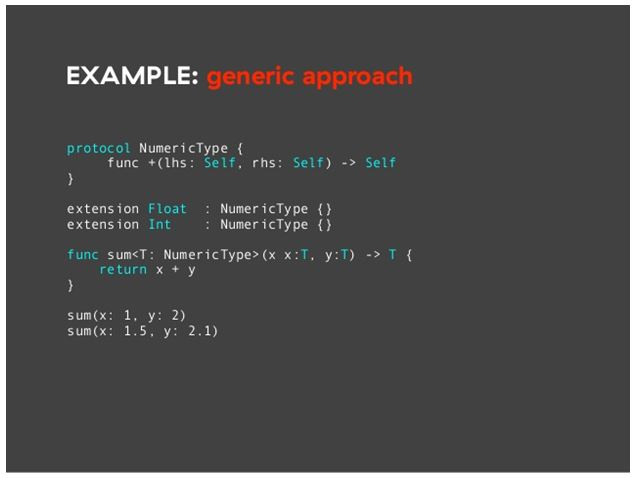## Swift – Generics

Generic helps us to write code which is flexible, reusable functions and types which have a different type like Int, float, string etc. Generic reduces the duplication of code, code with generic is represented in an abstract manner.

Generic is one of the new features that is present in the swift language.  In Swift language, Array & Dictionary type is both generic collections.  The array can be created with Int values, float values or an array with String values. Also, Dictionary can store values of any specified type, and there is no limit for what type of data is stored.

Below is a problem which Generics Solve:: –

This is a standard, a non-generic function called swap_TwoInts(_:_:), which swaps two Integer values:

1. func swap_TwoInts(_ a: inout Int, _ b: inout Int) {
2. let temporaryA = a
3. a = b
4. b = temporaryA
5. }

This function makes use of in-out parameters to swap the values of a and b.

The swap_TwoInts(_:_:) function swaps the original value of b with a, and the actual value of a with b. You can call this function to swap the values in two Int variables:

1. var someInt = 32
2. var anotherInt = 207
3. swap_TwoInts(&someInt, &anotherInt)
4. print(“someInteger is now \(someInt), and anotherInteger is now \(anotherInt)”)
5. // Prints “someInteger is now 207, and anotherInteger is now 32”

The swap_TwoInts(_:_:) function is useful, but it can only be used with Integer values. If you want to swap two String values, or two Double values, you have to write more functions, such as the swap_TwoStrings(_:_:) and swap_TwoDoubles(_:_:) functions shown below:

1. func swap_TwoStrings(_ a: in_out String, _ b: in_out String) {
2. let temporaryA = a
3. a = b
4. b = temporaryA
5. }
6. func swap_TwoDoubles(_ a: in_out Double, _ b: in_out Double) {
7. let temporaryA = a
8. a = b
9. b = temporaryA
10. }

You may have noticed that the bodies of the swap_TwoInts(_:_:), swap_TwoStrings(_:_:), and swap_TwoDoubles(_:_:) functions are identical. The only difference is the type of the values that they accept (Int, String, and Double).

If there is a single function which could swap two values of any type it would be more useful and flexible to use any type of value.

Note

If a and b are not of the same data type, it would not be possible to swap these values. Swift is a type-safe language, so it does not allow a variable of type string and variable of data type Double to swap values with one another. If we try to do so it will generate a compile-time error.### Generic Functions

Generic functions can work with any type of value. Here’s a generic version of the swap_TwoInts(_:_:) function from above, called swap_TwoValues(_:_:):

1. func swap_TwoValues<T>(_ a: inout T, _ b: inout T) {
2. let temporaryA = a
3. a = b
4. b = temporaryA
5. }

The body of the swap_TwoValues(_:_:) function is identical to the body of the swap_TwoInts(_:_:) function. However, the first line of swap_TwoValues(_:_:) is slightly different from swap_TwoInts(_:_:). Here’s how the first lines compare:

1. func swap_TwoInts(_ a: inout Int, _ b: inout Int)
2. func swap_TwoValues<T>(_ a: inout T, _ b: inout T)

The above example is generic the placeholder type name is called T instead of other types such as Int, Double and String. The placeholder type name (<T>)  doesn’t say about what T’s value type will be, but it does specify that both values a and b should of the same type T. Actual type of the T is determined when the function swap_TwoValues(_:_:) function is called.

To remember is one thing that the placeholder type name <T> is present inside the angular brackets. These brackets tell swift that T is a placeholder type name within the swap_twoValues(_:_:) function definition.

The other difference is that the generic function’s name (swap_TwoValues(_:_:)) is followed by the placeholder type name (T) inside angle brackets (<T>). The brackets tell Swift that T is a placeholder type name within the swap_TwoValues(_:_:) function definition. Because T is a placeholder, Swift _does not look for an actual type called T.

### Dinesh ChoudharyiOS Developer with 2+ years of experience.